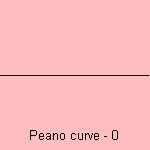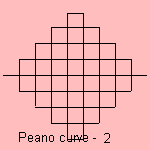# Peano curve

## fractal

last updated: 2005-01-07

In 1880 the Italian logician Giuseppe Peano (1858-1932) constructed the Peano curve, a base motif fractal which uses a line segment as base. The motif is dividing the line segment in three parts, and making a square up and down the middle part:This leads to a filled square, so the curve is a space-filling curve.

The fractal dimension of the Peano curve is equal to 2 1).
Later the name of Peano curve was given to any fractal whose fractal dimension is equal to 2.

notes

1) Fractal dimension = log N / log e, where N is the number of line segments and e the magnification.
For the Peano curve: N=9, e=3.